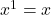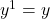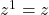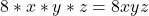Question

Question 2 (5 points)
Find the greatest common factor of 48xy3z, 24x2y3z, and 56x2yz.
A) 16xyz
B) 8xyz
C) 8xyz
D) XyZ

1.The greatest common factor is 8xyz

Step-by-step explanation:

To find the greatest common factor of an expression involving numbers and variables, we find each greatest common factor separately.

Numbers:

48, 24 and 56.

We find the greatest common factor factoring them simultaneously while all can be factored by the same number. The GCF is the multiplication of the factors. So

48 – 24 – 56|2

24 – 12 – 28|2

12 – 6 – 14|2

6 – 3 – 7|

They cant be factored by the same factors anymore, so the numeric GCF is 8.

Variables:

For each variable, the GCF will be the lowest exponent.

Variable x: We have exponents 1, 2 and 2. So the GCF isVariable y: We have exponents 3, 3 and 1. So the GCF isVariable z: We have exponents 1, 1 and 1. So the GCF isThe greatest common factor is: